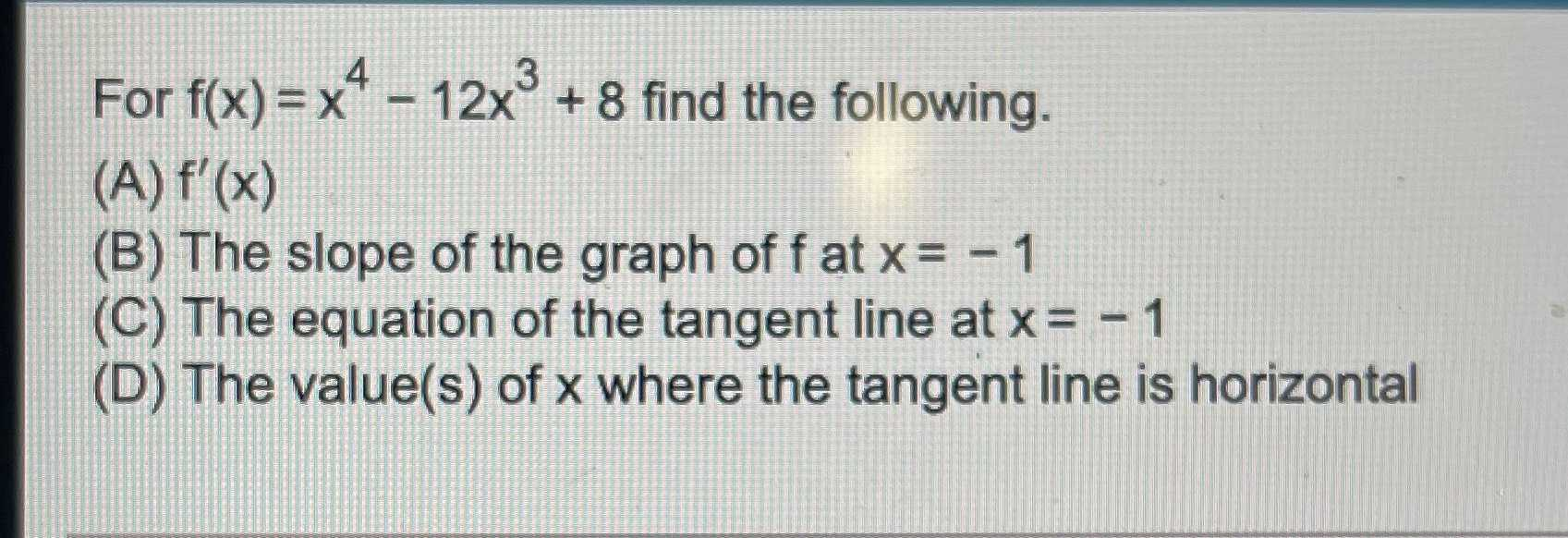### Still have math questions?

Algebra
QuestionFor $$f ( x ) = x ^ { 4 } - 12 x ^ { 3 } + 8$$ find the following.

(A) $$f ^ { \prime } ( x )$$

(B) The slope of the graph of $$f$$ at $$x = - 1$$

(C) The equation of the tangent line at $$x = - 1$$

(D) The value(s) of $$x$$ where the tangent line is horizontal

(A) $$f'(x)= 4x^3- 36x^2$$
(B) $$m= f'(- 1)= -40$$
(C) $$y= -40x- 19$$
(D) $$f'(x)= 0\Rightarrow x= 0,9$$